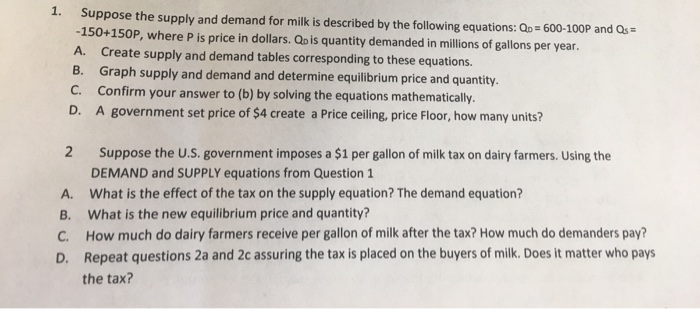### Create an Account

Home / Questions / 1. Suppose the supply and demand for milk is described by the following equations: Qp 600-100P and Q

# 1. Suppose the supply and demand for milk is described by the following equations: Qp 600-100P and Q1. Suppose the supply and demand for milk is described by the following equations: Qp 600-100P and Qs -150+150 P, where P is price in dollars. Qp is quantity demanded in millions of gallons per year. Create supply and demand tables corresponding to these equations. B. Graph supply and demand and determine equilibrium price and quantity. C. Confirm your answer to (b) by solving the equations mathematically. A. D. A government set price of \$4 create a Price ceiling, price Floor, how many units? 2 Suppose the U.S. government imposes a \$1 per gallon of milk tax on dairy farmers. Using the DEMAND and SUPPLY equations from Question 1 What is the effect of the tax on the supply equation? The demand equation? A. What is the new equilibrium price and quantity? C. How much do dairy farmers receive per gallon of milk after the tax? How much do demanders pay? D. Repeat questions 2a and 2c assuring the tax is placed on the buyers of milk. Does it matter who pays B. the tax?

Apr 06 2020 View more View LessSubscribe To Get Solution